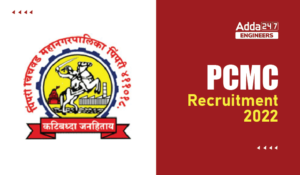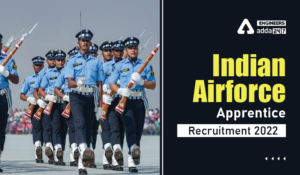Engineering Jobs   »   Quiz: Civil Engineering 23 March 2020

# Quiz: Civil Engineering 23 March 2020

Quiz: Civil Engineering
Exam: UPSSSC-JE
Topic: Hydraulics & Fluid Mechanics

Each question carries 1 mark
Negative marking: 1/3 mark
Time: 10 Minutes

Q1.The relationship between atmospheric pressure (Patm), gauge pressure (Pgauge) and absolute pressure (Pabs) is given by :
(a) Patm = Pabs + Pgauge
(b) Pabs = Patm + Pgauge
(c) Patm = Pabs + Pgauge
(d) none of the above
L1Difficulty 3
QTags Hydraulics & Fluid Mechanics
QCereator Anubhav Gupta

Q2. Wind speed is measured by an instrument
(a) Reflectometer
(b) Planimeter
(c) Anemometer
(d) Speedometer
L1Difficulty 3
QTags Hydraulics & Fluid Mechanics
QCereator Anubhav Gupta

Q3. Viscosity of water in comparison to mercury is :
(a) Higher
(b) Lower
(c) Same
(d) Higher/Lower depending on Temperature
L1Difficulty 3
QTags Hydraulics & Fluid Mechanics
QCereator Anubhav Gupta

Q4. Dynamic viscosity of a fluid with specific gravity 1.3 is measured to be 0.0034 Ns/m² its kinematic viscosity, in m²/sec is :
(a) 2.6 × 10^(–6)
(b) 4.4 × 10^(–6)
(c) 5.8 × 10^(–6)
(d) 7.2 × 10^(–6)
L1Difficulty 3
QTags Hydraulics & Fluid Mechanics
QCereator Anubhav Gupta

Q5. Poise is unit of
(a) (Dyne-sec⁡ )/(cm^2 )
(b) (Dyne-cm)/sec⁡〖 〗
(c) (Dyne-cm)/〖sec〗^2
(d) (Dyne-sec⁡ )/cm
L1Difficulty 3
QTags Hydraulics & Fluid Mechanics
QCereator Anubhav Gupta

Q6. The depth of centre of Pr. For a vertically immersed surface from the liquid surface given by :
(a) I_G/A_¯X – ¯X
(b) I_G/¯X – A_¯X
(c) A_¯X/I_G + ¯X
(d) I_G/A_¯X + ¯X
L1Difficulty 3
QTags Hydraulics & Fluid Mechanics
QCereator Anubhav Gupta

Q7. Which one of the following pressure unit represents the least pressure?
(a) Millibar
(b) mm of Hg
(c) N/mm²
(d) kgf/cm²
L1Difficulty 3
QTags Hydraulics & Fluid Mechanics
QCereator Anubhav Gupta

Q8. Atmospheric pressure is measured by _____ .
(a) Altimeter
(b) Piezometer
(c) Barometer
(d) Spectrometer
L1Difficulty 3
QTags Hydraulics & Fluid Mechanics
QCereator Anubhav Gupta

(a) (Wetted perimeter)/(Cross-sectional Area)
(b) (Cross-sectional Area)/(Wetted perimeter)
(d) All of above
L1Difficulty 3
QTags Hydraulics & Fluid Mechanics
QCereator Anubhav Gupta

Q10. Spherical shape of droplets of mercury is due to
(a) high density
(b) high surface tension
(d) Water
L1Difficulty 3
QTags Hydraulics & Fluid Mechanics
QCereator Anubhav Gupta

Solutions

S1. Ans.(b)
Sol: Pabs = Patm + Pgauge

S2. Ans.(c)

S3. Ans.(b)

S4. Ans.(a)
Sol. μ = 0.0034 Ns/m²
G = 1.3
γ = 3
γ = μ/ρ = μ/(Gγ_ω )
⇒ (0.0034 Ns/m^2 )/(1.3×1000 kg/m³)
⇒ 2.6 × 10^(–6) m²/sec.

S5. Ans.(a)

S6. Ans.(d)
Sol. ¯h = (I_G sin²θ)/(A¯X) + ¯X
For vertically θ = 90°
[¯h = (I_G sin²θ)/(A¯X) + ¯X]
S7. Ans.(a)
Sol. 1 millibar = 10^(–3) bar
= 10^(–3) × 10^5 N/m²
= 100 N/m²
1 mm of Hg = (1×13.6×1000×9.81)/1000
= 133.416 N/m²
1 N/mm² = 10^6 N/m²
1 kgf/cm² = (1×9.81 N)/10^(–4) = 9.81 × 10^4 N/m²

S8. Ans.(c)
Sol.
S9. Ans.(b)
Sol.
Hydraulic Radius = (C⁄s Area)/(Wetter Perimeter) ▭(R=A/P)
S10. Ans.(b)
Sol. due to the surface tension is in balance with the internal pressure and the droplet form into sphere which is the shape for minimum surface area.

Sharing is caring!

Thank You, Your details have been submitted we will get back to you.
•How to Prepare for SSC JE 2022 General A...
•GAIL Recruitment 2022, GAIL 282 Non Exec...
•MP Vyapam Sub Engineer Notification 2022...
•PCMC Recruitment 2022, Check here the de...
•BEL Recruitment 2022, Check here For 13 ...
•Indian Air Force Apprentice Recruitment ...